# NCERT Solutions for Class 11 Chemistry Some Basic Concepts of Chemistry Part 2## myCBSEguide App

CBSE, NCERT, JEE Main, NEET-UG, NDA, Exam Papers, Question Bank, NCERT Solutions, Exemplars, Revision Notes, Free Videos, MCQ Tests & more.

NCERT solutions for Class 11 Chemistry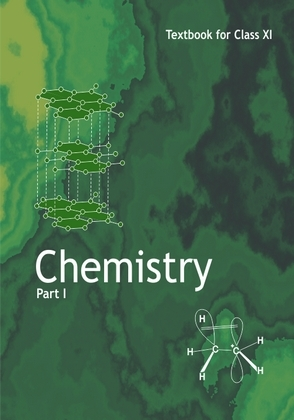## NCERT Class 11 Chemistry Chapter wise Solutions

• Chapter 1 – Some Basic Concepts of Chemistry
• Chapter 2 – Structure of Atom
• Chapter 3 – Classification of Elements and Periodicity in Properties
• Chapter 4 – Chemical Bonding and Molecular Structure
• Chapter 5 – States of Matter
• Chapter 6 – Thermodynamics
• Chapter 7 – Equilibrium
• Chapter 8 – Redox Reactions
• Chapter 9 – Hydrogen
• Chapter 10 – The s-Block Elements
• Chapter 11 – The p-Block Elements
• Chapter 12 – Organic Chemistry – Some Basic Principles and Techniques
• Chapter 13 – Hydrocarbons
• Chapter 14 – Environmental Chemistry

## NCERT Solutions for Class 11 Chemistry Some Basic Concepts of Chemistry Part 2

20. Round up the following upto three significant figures:

(i) 34.216

(ii) 10.4107

(iii) 0.04597

(iv) 2808

21. The following data are obtained when dinitrogen and dioxygen react together to form different compounds: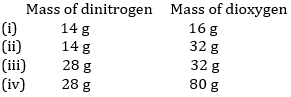(a) Which law of chemical combination is obeyed by the above experimental data? Give its statement.

(b) Fill in the blanks in the following conversions:

(i) 1 km = …………………. mm = …………………. pm

(ii) 1 mg = …………………. kg = …………………. ng

(iii) 1 mL = …………………. L = ………………….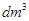22. If the speed of light is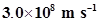, calculate the distance covered by light in 2.00 ns.

23. In a reaction A +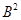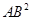Identify the limiting reagent, if any, in the following reaction mixtures.

(i) 300 atoms of A + 200 molecules of B

(ii) 2 mol A + 3 mol B

(iii) 100 atoms of A + 100 molecules of B

(iv) 5 mol A + 2.5 mol B

(v) 2.5 mol A + 5 mol B

24. Dinitrogen and dihydrogen react with each other to produce ammonia according to the following chemical equation: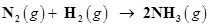(i) Calculate the mass of ammonia produced if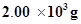dinitrogen reacts with 1.00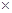103g of dihydrogen.

(ii) Will any of the two reactants remain unreacted?

(iii) If yes, which one and what would be its mass?

25. How are 0.50 mol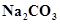and 0.50 Mdifferent?

26. If ten volumes of dihydrogen gas react with five volumes of dioxygen gas, how many volumes of water vapour would be produced?

27. Convert the following into basic units:

(i) 28.7 pm

(ii) 15.15 pm

(iii) 25365 mg

28. Which one of the following will have largest number of atoms?

(i) 1 g Au (s)

(ii) 1 g Na (s)

(iii) 1 g Li (s)

(iv) 1 g of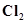(g)

29. Calculate the molarity of a solution of ethanol in water in which the mole fraction of ethanol is 0.040 (assume the density of water to be one).

30. What will be the mass of one 12C atom in g?

31. How many significant figures should be present in the answer of the following calculations?

(i)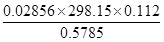(ii) 5 × 5.364

(iii) 0.0125 + 0.7864 + 0.0215

32. Use the data given in the following table to calculate the molar mass of naturally occurring argon isotopes: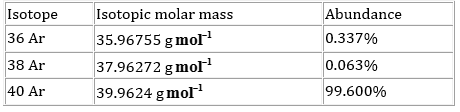33. Calculate the number of atoms in each of the following (i) 52 moles of Ar (ii) 52 u of He (iii) 52 g of He.

34. A welding fuel gas contains carbon and hydrogen only. Burning a small sample of it in oxygen gives 3.38 g carbon dioxide, 0.690 g of water and no other products. A volume of 10.0 L (measured at STP) of this welding gas is found to weigh 11.6 g. Calculate (i) empirical formula, (ii) molar mass of the gas, and (iii) molecular formula.

35. Calcium carbonate reacts with aqueous HCl to give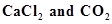according to the reaction,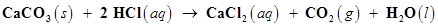What mass of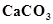is required to react completely with 25 mL of 0.75 M HCl?

36. Chlorine is prepared in the laboratory by treating manganese dioxide (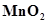) with aqueous hydrochloric acid according to the reaction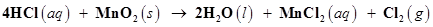How many grams of HCl react with 5.0 g of manganese dioxide?

## NCERT Solutions for Class 11 Chemistry Chapter 1 Some Basic Concepts of Chemistry

NCERT Solutions Class 11 Chemistry Some Basic Concepts of Chemistry Part 2 PDF (Download) Free from myCBSEguide app and myCBSEguide website. Ncert solution class 11 Chemistry includes text book solutions from both part 1 and part 2. NCERT Solutions for CBSE Class 11 Chemistry have total 14 chapters. 11 Chemistry NCERT Solutions in PDF for free Download on our website. Ncert Chemistry class 11 solutions PDF and Chemistry ncert class 11 PDF solutions with latest modifications and as per the latest CBSE syllabus are only available in myCBSEguide

## NCERT Solutions for Science Class 3rd to 12th

To download NCERT Solutions for class 11 Chemistry, Physics, Biology, History, Political Science, Economics, Geography, Computer Science, Home Science, Accountancy, Business Studies and Home Science; do check myCBSEguide app or website. myCBSEguide provides sample papers with solution, test papers for chapter-wise practice, NCERT solutions, NCERT Exemplar solutions, quick revision notes for ready reference, CBSE guess papers and CBSE important question papers. Sample Paper all are made available through the best app for CBSE students and myCBSEguide.com website.